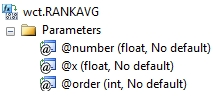# SQL Server RANK.AVG function

RANKAVG

Updated: 28 February 2011

Use the aggregate function RANKAVG to return the average rank of a number in a list of numbers. The rank of a number is its size relative to other values in a list. If more than one value has the same rank, the average is returned.
SyntaxArguments
@number
is the number whose rank you want to find. @number is an expression of type float or of a type that can be implicitly converted to float.
@x
the values to be used in the RANK calculation. @x is an expression of type float or of a type that can be implicitly converted to float.
@order
is a number specifying how to rank @number. If @order is zero or NULL, then RANK is calculated in descending order, Otherwise RANK is calculated in ascending order. @order is an expression of type float or of a type that can be implicitly converted to float.
Return Types
float
Remarks
·         RANKAVG gives duplicate numbers the same rank. However, the presence of duplicate numbers affects the ranks of subsequent numbers. For example, in a list of integers sorted in ascending order, if the number 10 appears twice and has a rank of 5, then 11 would have a rank of 7 (no number would have a rank of 6).
·         If @number is not contained in the dataset, RANKAVG returns an error.
·         @number must remain invariant for the GROUP.
·         @order must remain invariant for the group.
·         RANKAVG is an aggregate function and follows the same conventions as all other aggregate functions in SQL Server.
·         Available in XLeratorDB / statistics 2008 only
Examples
To ascertain where the number 2 ranks, from highest to lowest
SELECT wct.RANKAVG(
2     --@number
,x    --@x
,0    --@order
) as RANKAVG
FROM (VALUES
(1),(2),(2),(2),(2),(3),(4),(5),(6),(7),(8),
(8),(8),(8),(9),(10),(11),(12),(13),(13),(14)
) n(x)

This produces the following result.
RANKAVG
----------------------
18.5

(1 row(s) affected)

To ascertain where the number 2 ranks, from lowest to highest
SELECT wct.RANKAVG(2,x,1) as RANKAVG
FROM (VALUES
(1),(2),(2),(2),(2),(3),(4),(5),(6),(7),(8),
(8),(8),(8),(9),(10),(11),(12),(13),(13),(14)
) n(x)

This produces the following result.
RANKAVG
----------------------
3.5

(1 row(s) affected)

### SupportCopyright 2008-2021 Westclintech LLC         Privacy Policy        Terms of Service